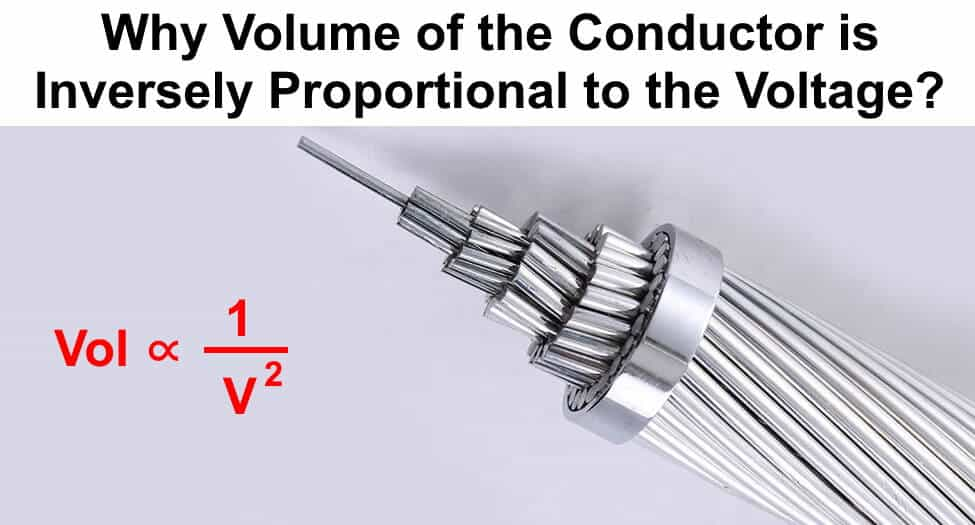# Why Volume of the Conductor is Inversely Proportional to the Square of Voltage?

Prove that the Volume of a conductor is inversely proportional to the squire of Voltage (Vol = 1/V2). And what is the effect of this relation on Transmission and Distribution System?Power in Three Phase Circuits = P = √3 V I Cosθ   OR     I = P / √3 V Cos θ            (1)

Law’s of resistance =                 R = ρ (l /A)                                                                (2)

Power Loss in Three Phase Circuits = P = 3 I2R                                                        (3)

Putting the values of current “I” and resistance “R” from equation (1) and (2) into equation (3).

P = 3 (P / √3 V Cos θ)2 x [ρ (l /A)]

P = 3 (P2 / 3 V2 Cosθ2) x ρ (l /A)

P = W = P2 ρ l / V2 Cosθ2 A … OR … A = P2 ρ l / V2 Cosθ2 W                                  (4)

Volume of the conductor in three phase system = Vol = 3 A x Length                        (5)

Putting the value of area “A” from Equation (4) into Equation (5)

Vol = 3 P2 ρ l / W V2 Cosθ2

Hence proved that volume of the conductor is inversely proportional to the squire of voltage

Volume of the Conductor = Vol ∝ 1 / V2

Effect of this relation ( Vol = 1 / V2 )  on Transmission and Distribution System

Actually, this relation has a very important rule in transmission and distribution system i.e. power system.

As we proved that volume of the conductor s inversely proportional to the squire of voltage, it means if we increase the level of voltage for transmission and distribution purpose, then volume of the conductor (size of the conductor) will reduce twice which is more effective approach to reduce the system cost. As more current leads to more problem due to high power rating, large size of the appliance, power factor problems, and more power losses etc.

In other words, if we use low level of the voltage for long transmission lines, the size of conductor will increased twice which leads to increase the overall cost of the system.

This is the exact reason that why we transmit power at high voltage and low current (when voltage increases then current decreases because generated power is constant).